# Bézier Surface (in 3D)

Written by Paul Bourke
December 1996

Contribution by Prashanth Udupa on Bezier Surfaces in VTK Designer 2: Bezier_VTKD2.pdf

The Bézier surface is formed as the Cartesian product of the blending functions of two orthogonal Bézier curves.Where Pi,j is the i,jth control point. There are Ni+1 and Nj+1 control points in the i and j directions respectively.

The corresponding properties of the Bézier curve apply to the Bézier surface.
- The surface does not in general pass through the control points except for the corners of the control point grid.
- The surface is contained within the convex hull of the control points.

Along the edges of the grid patch the Bézier surface matches that of a Bézier curve through the control points along that edge.Closed surfaces can be formed by setting the last control point equal to the first. If the tangents also match between the first two and last two control points then the closed surface will have first order continuity.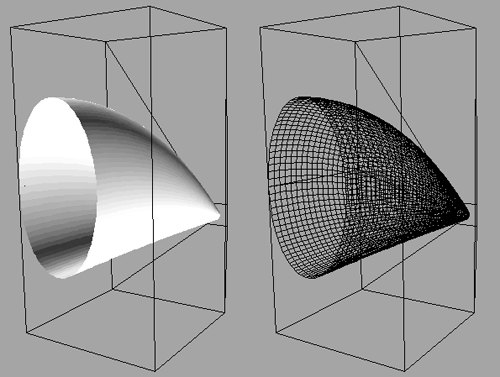While a cylinder/cone can be formed from a Bézier surface, it is not possible to form a sphere.

C Source Example

The following source code generates the surface shown in the first example above. It is provided for illustration only, the headers and prototype files are not given.

```#define NI 5
#define NJ 4
XYZ inp[NI+1][NJ+1];
#define RESOLUTIONI 10*NI
#define RESOLUTIONJ 10*NJ
XYZ outp[RESOLUTIONI][RESOLUTIONJ];

int main(argc,argv)
int argc;
char **argv;
{
int i,j,ki,kj;
double mui,muj,bi,bj;

/* Create a random surface */
srandom(1111);
for (i=0;i<=NI;i++) {
for (j=0;j<=NJ;j++) {
inp[i][j].x = i;
inp[i][j].y = j;
inp[i][j].z = (random() % 10000) / 5000.0 - 1;
}
}

for (i=0;i<RESOLUTIONI;i++) {
mui = i / (double)(RESOLUTIONI-1);
for (j=0;j<RESOLUTIONJ;j++) {
muj = j / (double)(RESOLUTIONJ-1);
outp[i][j].x = 0;
outp[i][j].y = 0;
outp[i][j].z = 0;
for (ki=0;ki<=NI;ki++) {
bi = BezierBlend(ki,mui,NI);
for (kj=0;kj<=NJ;kj++) {
bj = BezierBlend(kj,muj,NJ);
outp[i][j].x += (inp[ki][kj].x * bi * bj);
outp[i][j].y += (inp[ki][kj].y * bi * bj);
outp[i][j].z += (inp[ki][kj].z * bi * bj);
}
}
}
}

printf("LIST\n");

/* Display the surface, in this case in OOGL format for GeomView */
for (i=0;i<RESOLUTIONI-1;i++) {
for (j=0;j<RESOLUTIONJ-1;j++) {
printf("%g %g %g 1 1 1 1\n",
outp[i][j].x,    outp[i][j].y,    outp[i][j].z);
printf("%g %g %g 1 1 1 1\n",
outp[i][j+1].x,  outp[i][j+1].y,  outp[i][j+1].z);
printf("%g %g %g 1 1 1 1\n",
outp[i+1][j+1].x,outp[i+1][j+1].y,outp[i+1][j+1].z);
printf("%g %g %g 1 1 1 1\n",
outp[i+1][j].x,  outp[i+1][j].y,  outp[i+1][j].z);
}
}
printf("}\n");

/* Control point polygon */
for (i=0;i<NI;i++) {
for (j=0;j<NJ;j++) {
printf("{ = SKEL 4 1  \n");
printf("%g %g %g \n",inp[i][j].x,inp[i][j].y,inp[i][j].z);
printf("%g %g %g \n",inp[i][j+1].x,inp[i][j+1].y,inp[i][j+1].z);
printf("%g %g %g \n",inp[i+1][j+1].x,inp[i+1][j+1].y,inp[i+1][j+1].z);
printf("%g %g %g \n",inp[i+1][j].x,inp[i+1][j].y,inp[i+1][j].z);
printf("5 0 1 2 3 0\n");
printf("}\n");
}
}
} ```
Bézier Blending Function

This function computes the blending function as used in the Bézier surface code above. It is written for clarity, not efficiency. Normally, if the number of control points is constant, the blending function would be calculated once for each desired value of mu.

```double BezierBlend(k,mu,n)
int k;
double mu;
int n;
{
int nn,kn,nkn;
double blend=1;

nn = n;
kn = k;
nkn = n - k;

while (nn >= 1) {
blend *= nn;
nn--;
if (kn > 1) {
blend /= (double)kn;
kn--;
}
if (nkn > 1) {
blend /= (double)nkn;
nkn--;
}
}
if (k > 0)
blend *= pow(mu,(double)k);
if (n-k > 0)
blend *= pow(1-mu,(double)(n-k));

return(blend);
}```

# Bézier curves

Written by Paul Bourke
Original: April 1989, Updated: December 1996

The following describes the mathematics for the so called Bézier curve. It is attributed and named after a French engineer, Pierre Bézier, who used them for the body design of the Renault car in the 1970's. They have since obtained dominance in the typesetting industry and in particular with the Adobe Postscript and font products.

Consider N+1 control points pk (k=0 to N) in 3 space. The Bézier parametric curve function is of the formB(u) is a continuous function in 3 space defining the curve with N discrete control points Pk. u=0 at the first control point (k=0) and u=1 at the last control point (k=N).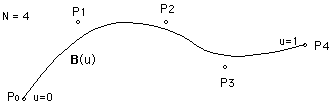Notes:

• The curve in general does not pass through any of the control points except the first and last. From the formula B(0) = P0 and B(1) = PN.

• The curve is always contained within the convex hull of the control points, it never oscillates wildly away from the control points.

• If there is only one control point P0, ie: N=0 then B(u) = P0 for all u.

• If there are only two control points P0 and P1, ie: N=1 then the formula reduces to a line segment between the two control points.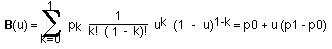• the term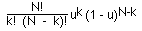is called a blending function since it blends the control points to form the Bézier curve.

• The blending function is always a polynomial one degree less than the number of control points. Thus 3 control points results in a parabola, 4 control points a cubic curve etc.

• Closed curves can be generated by making the last control point the same as the first control point. First order continuity can be achieved by ensuring the tangent between the first two points and the last two points are the same.

• Adding multiple control points at a single position in space will add more weight to that point "pulling" the Bézier curve towards it.• As the number of control points increases it is necessary to have higher order polynomials and possibly higher factorials. It is common therefore to piece together small sections of Bézier curves to form a longer curve. This also helps control local conditions, normally changing the position of one control point will affect the whole curve. Of course since the curve starts and ends at the first and last control point it is easy to physically match the sections. It is also possible to match the first derivative since the tangent at the ends is along the line between the two points at the end.
Second order continuity is generally not possible.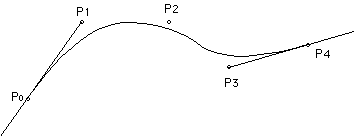• Except for the redundant cases of 2 control points (straight line), it is generally not possible to derive a Bézier curve that is parallel to another Bézier curve.

• A circle cannot be exactly represented with a Bézier curve.

• It isn't possible to create a Bézier curve that is parallel to another, except in the trivial cases of coincident parallel curves or straight line Bézier curves.

• Special case, 3 control points

B(u) = P0 * ( 1 - u ) 2 + P1 * 2 * u ( 1 - u ) + P2 u2

• Special case, 4 control points

B(u) = P0 * ( 1 - u )3 + P1 * 3 * u * ( 1 - u )2 + P2 * 3 * u2 * ( 1 - u ) + P3 * u3

Bézier curves have wide applications because they are easy to compute and very stable. There are similar formulations which are also called Bézier curves which behave differently, in particular it is possible to create a similar curve except that it passes through the control points. See also Spline curves.

Examples

The pink lines show the control point polygon, the grey lines the Bézier curve.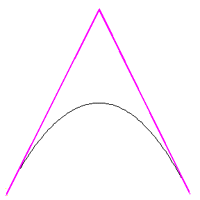The degree of the curve is one less than the number of control points, so it is a quadratic for 3 control points. It will always be symmetric for a symmetric control point arrangement.The curve always passes through the end points and is tangent to the line between the last two and first two control points. This permits ready piecing of multiple Bézier curves together with first order continuity.The curve always lies within the convex hull of the control points. Thus the curve is always "well behaved" and does not oscillating erratically.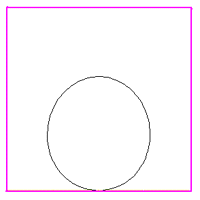Closed curves are generated by specifying the first point the same as the last point. If the tangents at the first and last points match then the curve will be closed with first order continuity.. In addition, the curve may be pulled towards a control point by specifying it multiple times.
C source

```/*
Three control point Bezier interpolation
mu ranges from 0 to 1, start to end of the curve
*/
XYZ Bezier3(XYZ p1,XYZ p2,XYZ p3,double mu)
{
double mum1,mum12,mu2;
XYZ p;

mu2 = mu * mu;
mum1 = 1 - mu;
mum12 = mum1 * mum1;
p.x = p1.x * mum12 + 2 * p2.x * mum1 * mu + p3.x * mu2;
p.y = p1.y * mum12 + 2 * p2.y * mum1 * mu + p3.y * mu2;
p.z = p1.z * mum12 + 2 * p2.z * mum1 * mu + p3.z * mu2;

return(p);
}

/*
Four control point Bezier interpolation
mu ranges from 0 to 1, start to end of curve
*/
XYZ Bezier4(XYZ p1,XYZ p2,XYZ p3,XYZ p4,double mu)
{
double mum1,mum13,mu3;
XYZ p;

mum1 = 1 - mu;
mum13 = mum1 * mum1 * mum1;
mu3 = mu * mu * mu;

p.x = mum13*p1.x + 3*mu*mum1*mum1*p2.x + 3*mu*mu*mum1*p3.x + mu3*p4.x;
p.y = mum13*p1.y + 3*mu*mum1*mum1*p2.y + 3*mu*mu*mum1*p3.y + mu3*p4.y;
p.z = mum13*p1.z + 3*mu*mum1*mum1*p2.z + 3*mu*mu*mum1*p3.z + mu3*p4.z;

return(p);
}

/*
General Bezier curve
Number of control points is n+1
0 <= mu < 1    IMPORTANT, the last point is not computed
*/
XYZ Bezier(XYZ *p,int n,double mu)
{
int k,kn,nn,nkn;
double blend,muk,munk;
XYZ b = {0.0,0.0,0.0};

muk = 1;
munk = pow(1-mu,(double)n);

for (k=0;k<=n;k++) {
nn = n;
kn = k;
nkn = n - k;
blend = muk * munk;
muk *= mu;
munk /= (1-mu);
while (nn >= 1) {
blend *= nn;
nn--;
if (kn > 1) {
blend /= (double)kn;
kn--;
}
if (nkn > 1) {
blend /= (double)nkn;
nkn--;
}
}
b.x += p[k].x * blend;
b.y += p[k].y * blend;
b.z += p[k].z * blend;
}

return(b);
}
```

# Piecewise Cubic Bézier Curves

Written by Paul Bourke
March 2000

Given four points p0, p1, p2, and p3 in 3D space the cubic Bézier curve is defined as

p(t) = a t3 + b t2 + c t + p0

where t ranges from 0 (the start of the curve, p0) to 1 (the end of the curve, p3). The vectors a, b, c are given as follows:

c = 3 (p1 - p0)

b = 3 (p2 - p1) - c

a = p3 - p0 - c - b

In the following examples the green markers correspond to p0 and p3 of each section. The blue markers correspond to p1 and p2. The grey curve is the Bézier curve sampled 20 times, the samples are shown in red. The coordinates for each vertex is shown on the right.

Example 1
This is a single minimum piece of a piecewise Bézier curve. It is defined by 4 points, the curve passes through the two end points. The tangent at the end points is along the line to the middle two points.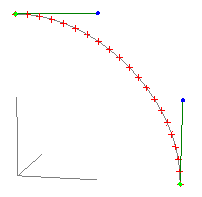0 0 10.5 0 11 0 0.51 0 0

Example 2
Multiple curve pieces can be joined together to form longer continuous curves. The curve is made continuous by the setting the tangents the same at the join. Note that each piece of the curve is defined by t ranging from 0 to 1.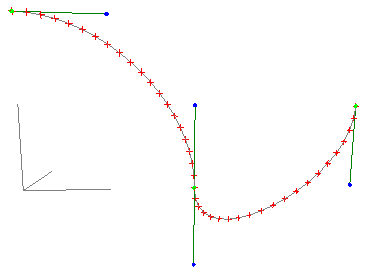0 0 10.5 0 11 0 0.51 0 0 1 0 01 0 -0.52 0 02 0 0.5

Example 3
By changing the tangent points between two curve pieces, sharp transitions can be created.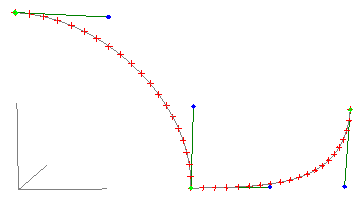0 0 10.5 0 11 0 0.51 0 0 1 0 01.5 0 02 0 02 0 0.5

Example 4
The "strength" at the end points is controlled by the length of the tangent lines. The longer the line the more effect that tangent has. If the curve is being used for animation steps then the strength also controls the velocity, note the samples shown in red are further apart for the long tangent vectors.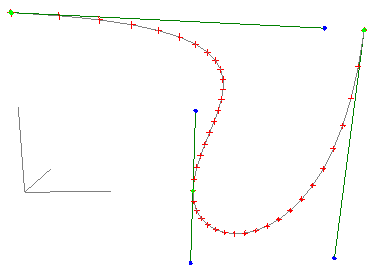0 0 11.75 0 11 0 0.51 0 0 1 0 01 0 -0.52 0 -0.52 0 1

Example 5
Straight line geometry can readily be made by aligning the tangent vectors along the line. While this may seem a frivolous use, it can be put to good effect in animations applications. By adjusting the tangent points p1 and p2 the velocity along the line can be controlled.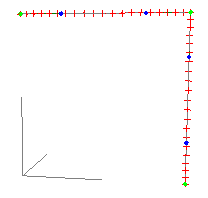0 0 10.25 0 10.75 0 11 0 1 1 0 11 0 0.751 0 0.251 0 0

Notes

• Piecewise cubic Bézier curves like their most general Bézier counterparts cannot exactly represent a circle.

• Except in the trivial case of a straight line, it isn't possible to create a Bézier curve that is parallel to another.

• There is no closed solution to finding the closest point on a Bézier curve to another point. The usual method is some kind of subdivision of t until some error tolerance is met.

Source code

```/*
Piecewise cubic bezier curve as defined by Adobe in Postscript
The two end points are p0 and p3
Their associated control points are p1 and p2
*/
XYZ CubicBezier(XYZ p0,XYZ p1,XYZ p2,XYZ p3,double mu)
{
XYZ a,b,c,p;

c.x = 3 * (p1.x - p0.x);
c.y = 3 * (p1.y - p0.y);
c.z = 3 * (p1.z - p0.z);
b.x = 3 * (p2.x - p1.x) - c.x;
b.y = 3 * (p2.y - p1.y) - c.y;
b.z = 3 * (p2.z - p1.z) - c.z;
a.x = p3.x - p0.x - c.x - b.x;
a.y = p3.y - p0.y - c.y - b.y;
a.z = p3.z - p0.z - c.z - b.z;

p.x = a.x * mu * mu * mu + b.x * mu * mu + c.x * mu + p0.x;
p.y = a.y * mu * mu * mu + b.y * mu * mu + c.y * mu + p0.y;
p.z = a.z * mu * mu * mu + b.z * mu * mu + c.z * mu + p0.z;

return(p);
}
```

FAQ

A common application for these curves in computer graphics is the creation of a smooth flight path that passes through keyframe points in space. The basic issue is how to derive the tangent vectors for each piece of the curve. There are two ways one might achieve this that are illustrated in the drawings below. The first approach is easiest but often lends to unnecessary "swerving", the second method is "smoother". In what follows the keyframes and points p0 to p5, in order to use the Piecewise Cubic Bézier for each section (between points pi and pi+1) one needs to find the tangent vectors shown in red. Note that for continuity between the points the tangent vector at the end of one piece is the negative of the tangent at the start of the next piece.

In this first case the tangent vectors are just the differences between subsequent keyframe points. So for example, for the segment between p1 and p2 the four points use for the Bézier would be p1, p2, 2p2-p3, p2. Depending on the length scaling for the tangent vectors, the resulting Bézier curve between points p1 and p3 is shown in blue.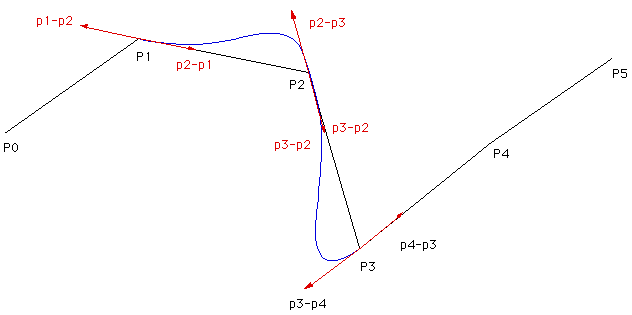A generally better method is shown below, again one needs to find the red tangent vectors. The exact implementation will be left up to the reader but the approach I've used is to find the cross product between the vectors to each neighbour, that is a vector coming out of the page (or into the page) in the diagram below. The tangent vectors (red) are found by taking the cross product of that with the green normal vectors. The main reason for using this approach is that it overcomes a mirror symmetry problem that occurs if one simple tries to rotate the green normal vectors +- 90 degrees. Note that the case of 3 collinear points needs to be treated as a special case.An improvement by Lars Jensen is illustrated below. It uses a normal that bisects the two vectors to the neighbouring points along with way of limiting the tangent lengths.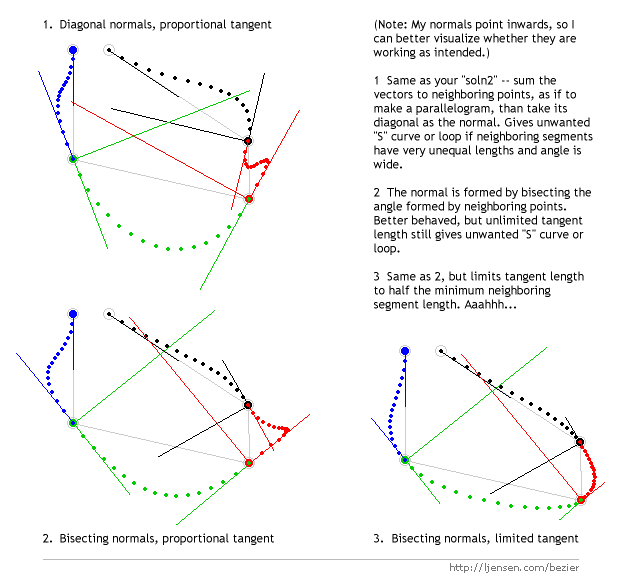The only remaining comment is how one deals with the first and last point, normally there are some ad hoc approaches that are application specific.